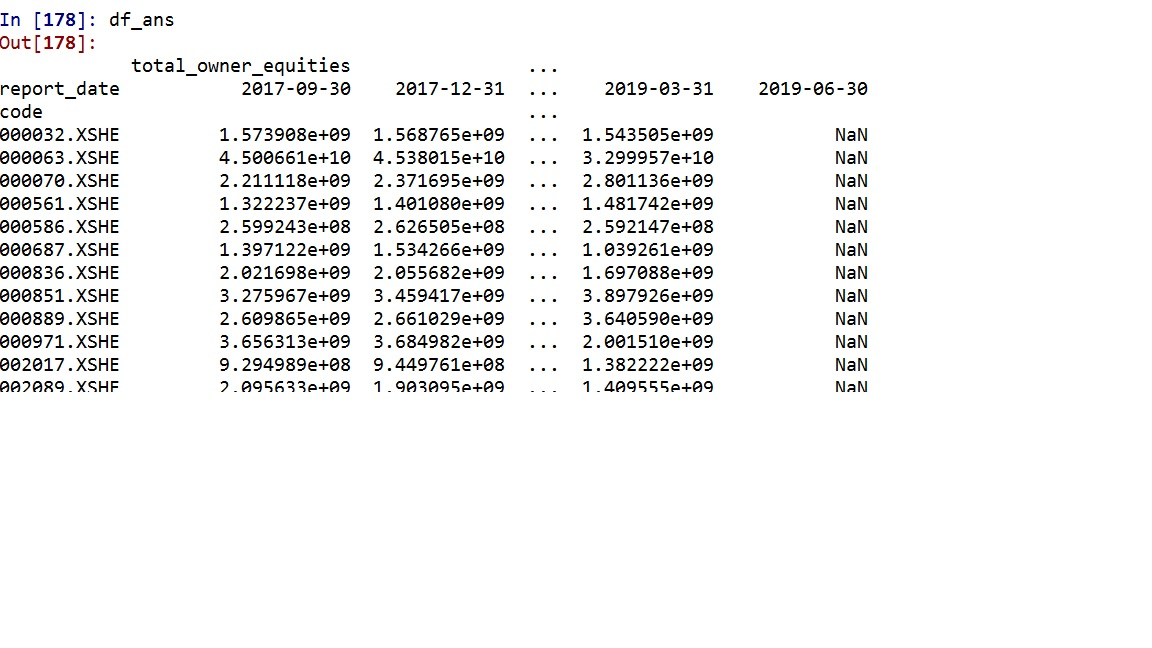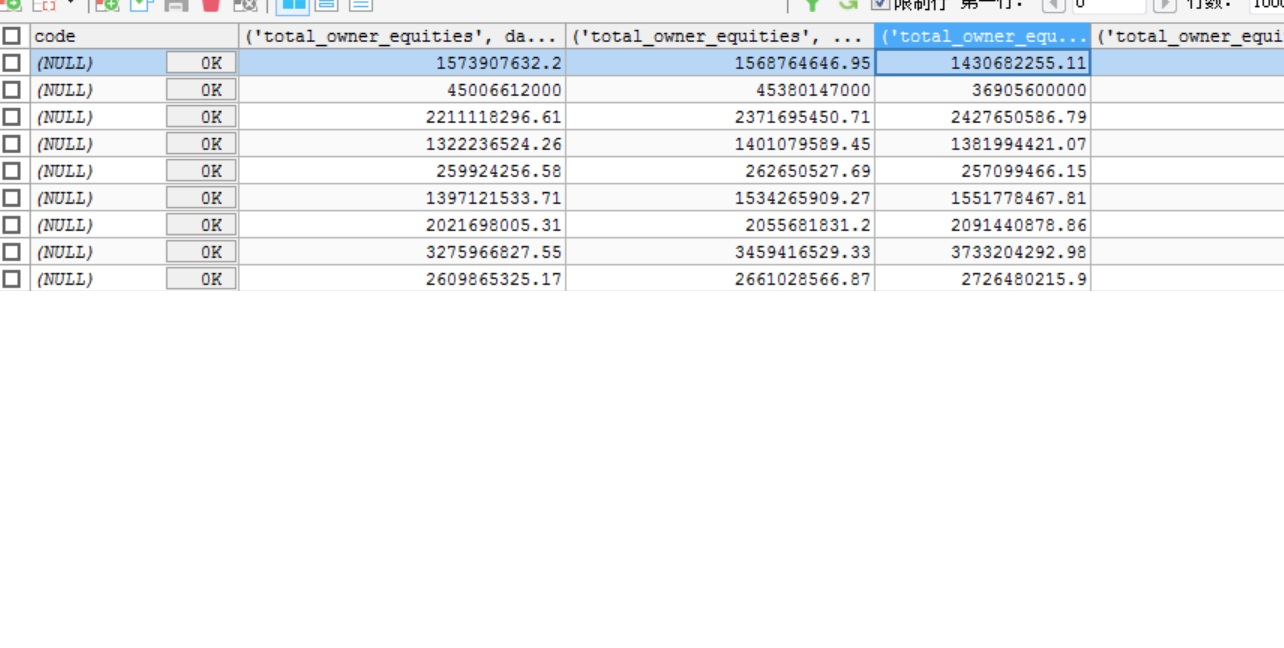dataframe.to_sql过程中遇到的索引问题engine = create_engine('mysql+pymysql://root:root@localhost:3306/test')

df_ans.to_sql(name='toe_list05', con=engine, if_exists='append',index = True,index_label=None)python里将dataframe转成matrix后，为什么参数会变

data=pd.DataFrame(data_train) data_matrix=data.as_matrix() dataframe输出的： Diabetes 8.091600 0.041000 matrix输出的 ['Diabetes' 8.0916 0.040999999999999995] 为什么0.041000会变成0.040999999999999995？

python pandas to_excel为什么会自动删除其他sheet

df = pandas.read_excel('test.xlsx') df = pandas.pivot_table(df, index=['a'], columns=['b'], aggfunc=numpy.sum) excelWriter=pandas.ExcelWriter('test_new.xlsx')--test_new.xlsx是一个已经存在的excel文件，有两个sheet1、sheet2 df.to_excel(excelWriter, sheet_name='Sheet1') excelWriter.save() 执行完之后，之前test_new.xlsx里的Sheet2为什么没有了，怎么才能保留

DataFrame中怎么转换为values不带中括号的字典形式？利用Geo画城市地图遇到困难了

Pasdas eval()和DataFrame.eval()真的可以提升性能？

# Pasdas eval()和DataFrame.eval()真的可以提升性能？ 测试代码：  nrows,ncols = 100000,100 rng = np.random.RandomState(42) df1,df2,df3,df4 = (pd.DataFrame(rng.rand(nrows,ncols)) for i in range(4)) %timeit (df1<df3) & (df2<1) | (df3<df4) %timeit pd.eval('(df1<df3) & (df2<1) | (df3<df4)')  测试结果： ![图片说明](https://img-ask.csdn.net/upload/202005/02/1588388630_853049.png) 对比下，运行时间并没有像网上那样提升那没多？这是因为电脑配置导致？

pandas绘制时间序列时x轴标签显示格式问题

ax1.xaxis.set_major_formatter(mdate.DateFormatter('%Y-%m-%d'))#设置时间标签显示格式 运行到这里时报错： module 'matpltlib.dates' has no attribute 'DateFormatter  完整代码如下：  #画时间曲线的函数 import matplotlib.dates as mdate from pylab import mpl mpl.rcParams['font.sans-serif'] = ['FangSong'] mpl.rcParams['axes.unicode_minus'] = False fig1 = plt.figure(figsize=(15,5)) ax1=fig1.add_subplot(111) fig1.set_size_inches(20, 4) ax1.xaxis.set_major_formatter(mdate.DateFormatter('%Y-%m-%d %H:%M'))#设置时间标签显示格式 plt.xticks(pd.date_range('2017-06-01','2017-06-30'),rotation=90)#设置时间标签显示格式 plt.plot(zhangsan["刷卡时间"],zhangsan["地点"],linewidth=3,color='r',marker='o', markerfacecolor='blue',markersize=12) plt.xlabel('time') plt.ylabel('position') plt.title('Single card punching in June') plt.savefig('E:/workplace/data/test2.jpg',dpi=400,bbox_inches='tight') plt.show() 

import pandas as pd from selenium import webdriver import re #设置无界面浏览器模式，不弹出模拟浏览器窗口，让程序在后台运行 chrome_options = webdriver.ChromeOptions() chrome_options.add_argument('--headless') browser = webdriver.Chrome(options=chrome_options) data_all = pd.DataFrame() # 创建一个空列表用来汇总所有的表格数据 for pg in range(1,60): # 可以将页码数调小进行快速尝试 url = 'http://yanbao.stock.hexun.com/ybsj5_' + str(pg)+ '.shtml' browser.get(url) # 通过Selenium库访问网页 data = browser.page_source # 获取网页源码 table = pd.read_html(data) # 通过pandas库提取表格数据 #print(table) # 添加股票代码 p_code = '<a href="yb_(.*?).shtml' code = re.findall(p_code, data) # print(code) # print(len(table)) # print(len(code)) # print(table['股票名称']) table['股票代码'] = code # 通过concat()函数将各页的表格纵向拼接成一个总的DataFrame data_all = pd.concat([data_all, table], join='outer', ignore_index=True) data_all.to_excel('分析师评级报告.xlsx', index=False) # 访问网页并获取网页源代码 url = 'http://yanbao.stock.hexun.com/ybsj.aspx?type=5' browser.get(url) data = browser.page_source ![图片说明](https://img-ask.csdn.net/upload/202004/07/1586246101_875401.jpg)

python 中pandas的value_counts是怎么算的？

from matplotlib.dates import DateFormatter from matplotlib.dates import DayLocator from matplotlib.dates import MonthLocator from matplotlib.dates import YearLocator from matplotlib.finance import _quotes_historical_yahoo from matplotlib.finance import _candlestick import pandas as pd from pandas import DataFrame as df from pandas import Series as sr import os import numpy as np os.chdir("D:\\python1") from datetime import date import matplotlib.pyplot as plt day_start = date (2011,11,4) day_end = date(2011,12,4) years =YearLocator() months = MonthLocator() days = DayLocator() year_formatter = DateFormatter("%b %Y") SP = _quotes_historical_yahoo('^GSPC',day_start,day_end) np.savetxt("SP.txt",SP,delimiter=',',newline='\n') SSE = _quotes_historical_yahoo('000001.SS',day_start,day_end) np.savetxt("SSE.txt",SSE,delimiter=',',newline='\n') fig = plt.figure() ax = fig.add_subplot(1,1,1) ax.xaxis.set_major_locator(years) ax.xaxis.set_minor_locator(months) ax.xaxis.set_major_formatter(year_formatter) _candlestick(ax,(SP,SSE)) fig.autofmt_xdate() plt.show()

 import pycountry_convert as pc def country_to_continent(country_name): country_alpha2=pc.country_name_to_country_alpha2(country_name) country_continent_code=pc.country_alpha2_to_continent_code(country_alpha2) country_continent_nam=pc.convert_continent_code_to_continent_name(country_continent_code) df_covid19['continent']=df_covid19.Country_Region.map(lambda x: country_to_continent(x)) ` 错误提示：TypeError: argument of type 'functools._lru_cache_wrapper' is not iterable 谁能帮我看看什么原因？

class myDataframe(pd.Dataframe) def __init__..... .... self.my_attribute = ['a', 'b', 'c'] 如果这么干的话，pandas会提示UserWarning: Pandas doesn't allow columns to be created via a new attribute name - see https://pandas.pydata.org/pandas-docs/stable/indexing.html#attribute-access 有咩有什么办法可以避免这个warning咧？ stackoverflow 上也有人问： https://stackoverflow.com/questions/47631230/pandas-store-a-list-as-a-dataframe-attribute 谢谢

python pd.merge()合并后的DataFrame无法输出到excel表

import numpy as np df2 = pd.DataFrame({'a':[7,8,9], 'b':[6,5,4]}, columns=['a', 'b']) df2.to_csv('a.csv', mode='a',header=False, index=False,sheet_name = 'r3frg') 返回错误TypeError: to_csv() got an unexpected keyword argument 'sheet_name'，按理说sheet_name不是自带的参数么，为什么失败了呢，a.csv存储路径也没有问题

Java基础知识面试题（2020最新版）

String s = new String(" a ") 到底产生几个对象？

Linux面试题（2020最新版）

Linux命令学习神器！命令看不懂直接给你解释！

loonggg读完需要3分钟速读仅需 1 分钟大家好，我是你们的校长。我之前讲过，这年头，只要肯动脑，肯行动，程序员凭借自己的技术，赚钱的方式还是有很多种的。仅仅靠在公司出卖自己的劳动时...

85后蒋凡：28岁实现财务自由、34岁成为阿里万亿电商帝国双掌门，他的人生底层逻辑是什么？...

MySQL数据库面试题（2020最新版）